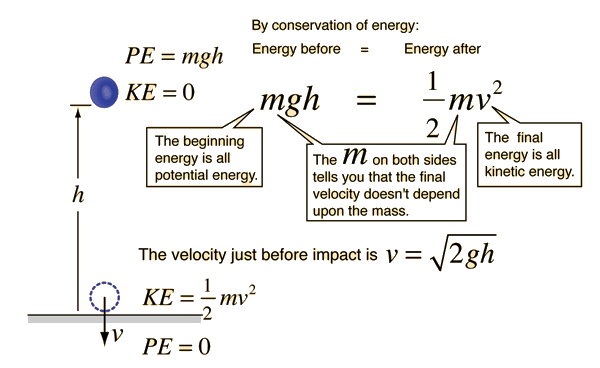# Energy as a tool for mechanics problem solving

The application of the conservation of energy principle provides a powerful tool for problem solving. Newton's laws are used for the solution of many standard problems, but often there are methods using energy which are more straightforward. For example, the solution for the impact velocity of a falling object is much easier by energy methods. The basic reason for the advantage of the energy approach is that just the beginning and ending energies need be considered; intermediate processes do not need to be examined in detail since conservation of energy gaurantees that the final energy of the system is the same as the initial energy.

The work-energy principle is also a useful approach to the use of conservation of energy in mechanics problem solving. It is particularly useful in cases where an object is brought to rest as in a car crash or the normal stopping of an automobile.

Index

 HyperPhysics***** Mechanics R Nave
Go Back

# Object Falling from Rest

As an object falls from rest, its gravitational potential energy is converted to kinetic energy. Conservation of energy as a tool permits the calculation of the velocity just before it hits the surface.If an object is dropped from height h = m, then the velocity just before impact is
v = m/s. If the mass is m = kg, then the kinetic energy just before impact is equal to
K.E. = J, which is of course equal to its initial potential energy. The accuracy of this calculation depends upon the assumption that air friction is negligible, and that the height of drop is small compared to the radius of the earth.

### Since you know velocity, mass, and kinetic energy, can you predict the force of impact?

Index

 HyperPhysics***** Mechanics R Nave
Go Back